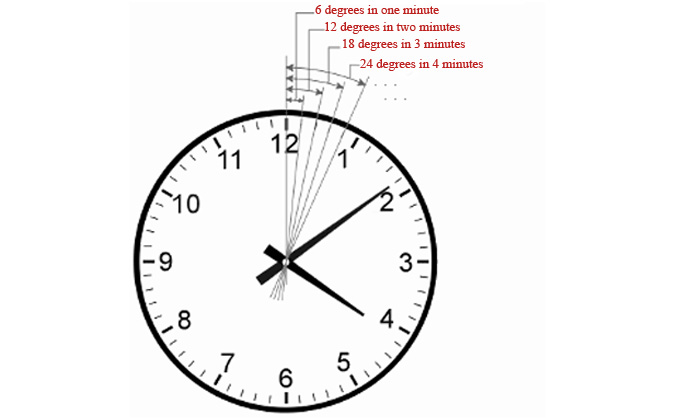×The Free 30-Day CAT RC Course

"It is designed to help you excel in the upcoming CAT 2023 exam"

-By Lavleen Kaur Kapoor. Over 1,73,000 Subscribers

No thanks >

OPEN

OPEN

## IBSAT, CAT, XAT, NMAT, GMAT

OPEN#### Get Personalized MBA Mentorship

Connect with my team on WhatsApp for unbiased MBA College Selection advice.

# QA Study material - Important Concepts and Formulas on Clock ProblemsCAT Registration Ends: 13th September 2023The QA (Quantitative Ability) section in the CAT 2022 will have 34 questions with 60 minutes sectional time limit. Clock problems were popular until a few years, but CAT 2022 may always surprise you.

Let us go through the Important Clock Formula, Concepts And Related Clock Questions that could be asked in the CAT 2022 exam.

## Clock Problems

The different types of clock questions that can be asked in the CAT 2022 exam are:

1. Angle related clock problems

2. Faulty Clock questions

3. Time gain or Time Loss clock questionsam Syllabus

## Clock Problem Concepts:

1. Minute Space: The circumference of the face or dial of the clock can be divided into 60 equal parts called minute space

2. A clock has two hands:

1. Smaller one is called the hour hand or short hand

2. Larger one is called minute hand or long hand.

3. Minute Gain: Every 60 minutes, the minute hand gains 55 minutes on the hour on the hour hand

4. Overlap: In every hour, both the hands coincide once.

5. Straight Line: The hands are in the same straight line when they are coincident or opposite to each other.

6. Clock Too Fast: If a watch or a clock indicates 8.15, when the correct time is 8, it is said to be 15 minutes too fast.

7. Clock Too Slow: if a clock indicates 45, when the correct time is 8, it is said to be 15 minutes too slow.

## Clock Formula:

1. 60-minute space = 360° = 1 hour

2. 1-minute space = 6° = 1 minute

3. 5-minute space = 6° x 5 = 30° = 5 minutes

4. Right Angle or Perpendicular = 15-minute spaces apart

5. Right Angle or Perpendicular = 22 times in 12 hours or 44 times in 24 hours (1 day)

6. Straight Angle or Straight Line or 180° = 30-minute space apart

7. Straight Angle = 11 times in 12 hours or 22 times in 24 hours (1 day)

8. Angle traced by hour hand in 12 hrs = 360°

9. Angle traced by minute hand in 60 min. = 360°

10. Speed of hour hand = 0.5 dpm (degree per minute)

11. Speed of minute hand = 6 dpm

12. Angle of the hour hand from vertical at N o’clock = 30N

## Illustrations:

Q) Find the angle between the minute hand and hour hand of a clock when the time is 7.20?
Ans: 100deg.

Sol:

Angle traced by the hour hand in 12 hours = 360 degrees.
• Angle traced by it in 7 hrs. 20 min i.e. 22/3 hrs. = [(360/12) * (22/3)] = 220 degrees
• Angle traced by minute hand in 60 min = 360 deg.
• Angle traced by it in 20 min = [(360/20) * 60] = 120 deg.
• Therefore, required angle = (220 - 120) = 100deg.

Q) The minute hand of a clock overtakes the hours hand at intervals of 65 min of the correct time. How much of the day does the clock gain or lose?

Ans: the clock gains 10 10/43 minutes

Sol:

In a correct clock, the minute hand gains 55 min. spaces over the hour hand in 60 minutes. To be together again, the minute hand must gain 60 minutes over the hour hand.
• 55 minutes are gained in 60 min.
• 60 min. are gained in [(60/55) * 60] min = 65 5/11 min.
• But they are together after 65 min.
• Therefore, gain in 65 minutes = (65 5/11 - 65) = 5/11 min.
• Gain in 24 hours = [(5/11) * (60*24)/65] = 10 10/43 min.
• Therefore, the clock gains 10 10/43 minutes in 24 hours.

Read More : Tricky Questions on Banker's Discount

Q) A clock is set right at 8 a.m. The clock gains 10 minutes in 24 hours. What will be the true time when the clock indicates 1 p.m. on the following day?

Ans. 48 min. past 12.

Sol:

Time from 8 a.m. on a day to 1 p.m. on the following day = 29 hours.
• 24 hours 10 min. of this clock = 24 hours of the correct clock.
• 145/6 hrs of this clock = 24 hours of the correct clock.
• 29 hours of this clock = [24 * (6/145) * 29] hrs of the correct clock
• = 28 hrs 48 min of the correct clock.
• Therefore, the correct time is 28 hrs 48 min. after 8 a.m.
• This is 48 min. past 12.

Q) At what time between 2 and 3 O’ clock will the hands of a clock together?

Ans: 10 10/11 min. past 2.

Sol:

At 2 O’ clock, the hour hand is at 2 and the minute hand is at 12, i.e. they are 10 min space apart. To be together, the minute hand must gain 10 minutes over the other hand. Now, 55 minutes are gained by it in 60 min.
• Therefore, 10 min will be gained in [(60/55) * 10] min = 10 10/11 min.
• Therefore, the hands will coincide at 10 10/11 min. past 2.

Complete Study Material on QA

Quantitative Ability - Geometry

Get Complete Information on CAT Exam and Syllabus.

Stay informed, Stay ahead and stay inspired with MBA Rendezvous